# Dog Simulator

## Description

The goal of this project was to implement a spherical, procedurally generated world that the user could navigate. The final product was not as polished as intended, but still satisfies these basic goals. It also includes procedurally generated obstacles and some minor collision detection based on bounding spheres.

## Controls

You can use the usual WASD keys to move the dog around the world.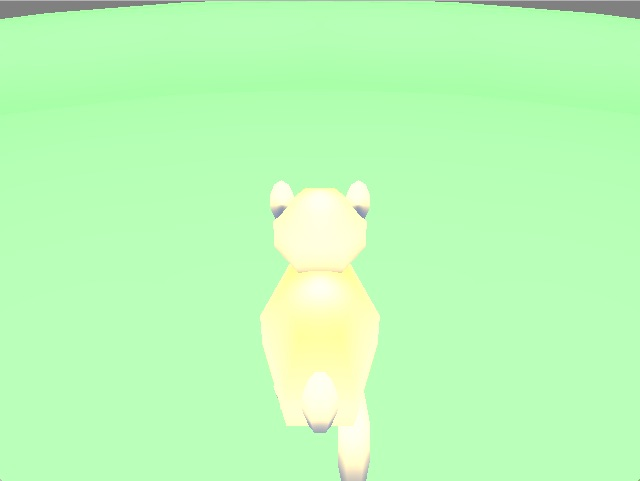Forward: W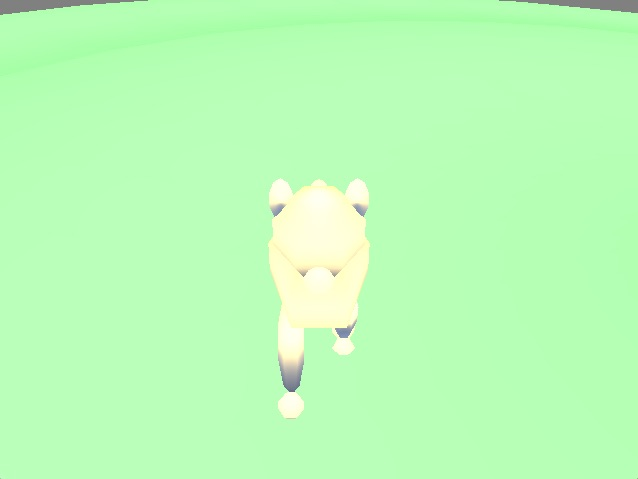Back: S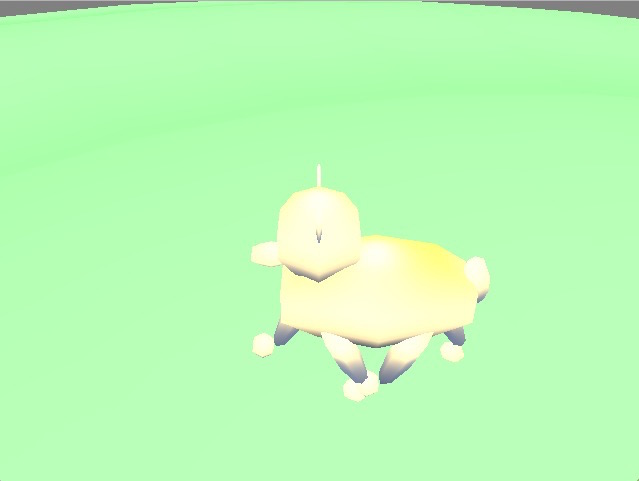Left: A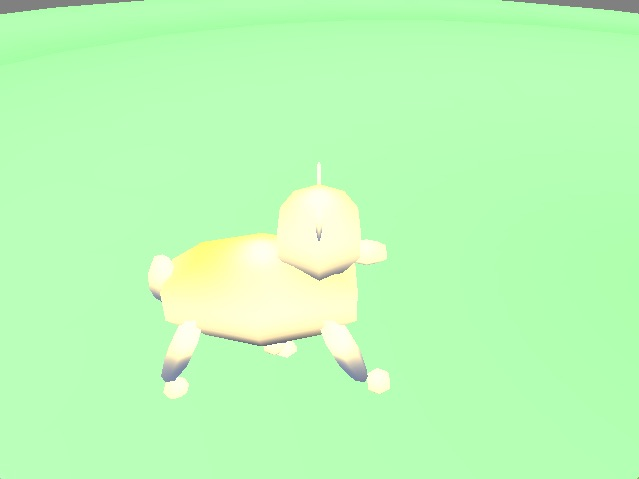Right: D

## Math

The first attempt at this project was a modification of the game camera we did in class. To make the camera move around a spherical world rather than a planar one, I used conversions from cartesian coordinates to spherical coordinates when setting the eye position.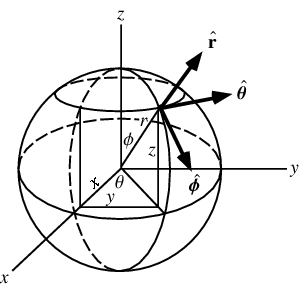Image from Wolfram MathWorld

x= radius * sin(phi) * cos(theta)

y = radius * sin(phi) * sin(theta)

z = radius * cos(phi)

Phi is the angle from the z axis while theta is the angle from the x axis. Radius is the radius of the spherical world, plus a small distance to keep the camera slight above the surface of the sphere. Using this conversion, I was able to change the position of the camera's eye to keep it on the surface of the sphere.

The difficulty unfortunately presented itself in how to create the look at point for the camera, then. Using only cartesian coordinates for it would not be convenient because the look at point needs to similarly follow the sphere's surface. I decided to simplify it by limiting the camera to rotating only around horizontally, i.e. around the up vector. However, I could not find the proper fomula to do this; the closest I was able to get was by keeping track of the angle of rotation:

gazePhi = sin(angle)

gazeTheta = cos(angle)

I then set the look at point based on the gaze vector:

lookAtX = radius * sin(totalPhi + gazePhi) * cos(totalTheta + gazeTheta)

lookAtY = radius * sin(totalPhi + gazePhi) * sin(totalTheta + gazeTheta)

lookAtZ = radius * cos(totalPhi + gazePhi)

The intent was to move the camera along the gaze vector with the WASD keys, but somewhere I went wrong with the math, because using these calculations caused the camera to move through the look at point and not stay on the surface of the sphere.

The current version of this program instead rotates the model of the world underneath the dog rather than moving the camera.

## Lessons Learned

- Using spherical coordinates rather than cartesian coordinates does not work if it is only applied to one element of a program. Though build-in functions like lookAt take cartesian coordinates, keeping calculations within the same coordinate system makes everything far easier to comprehend.

- Research is important. Look up how something can be done before picking it for a project.

- If something is going to be in color, make sure the screen you test it on is at a decent level of brightness to show what things will look like on other computers.